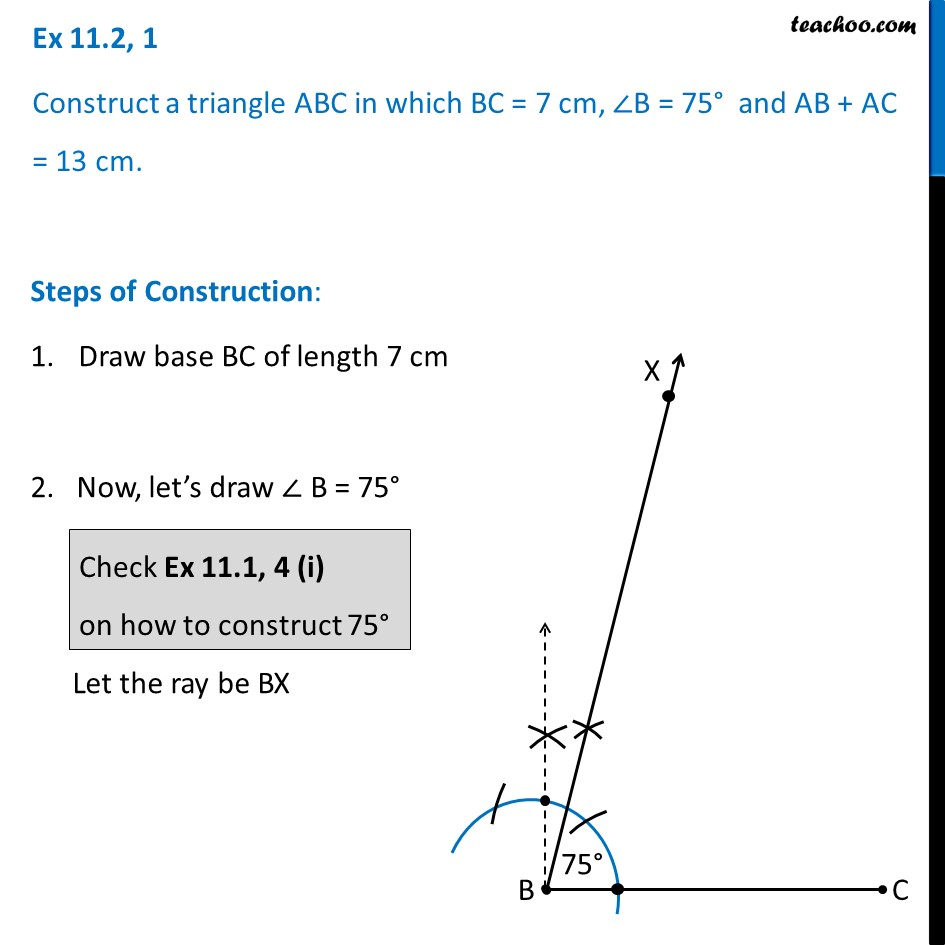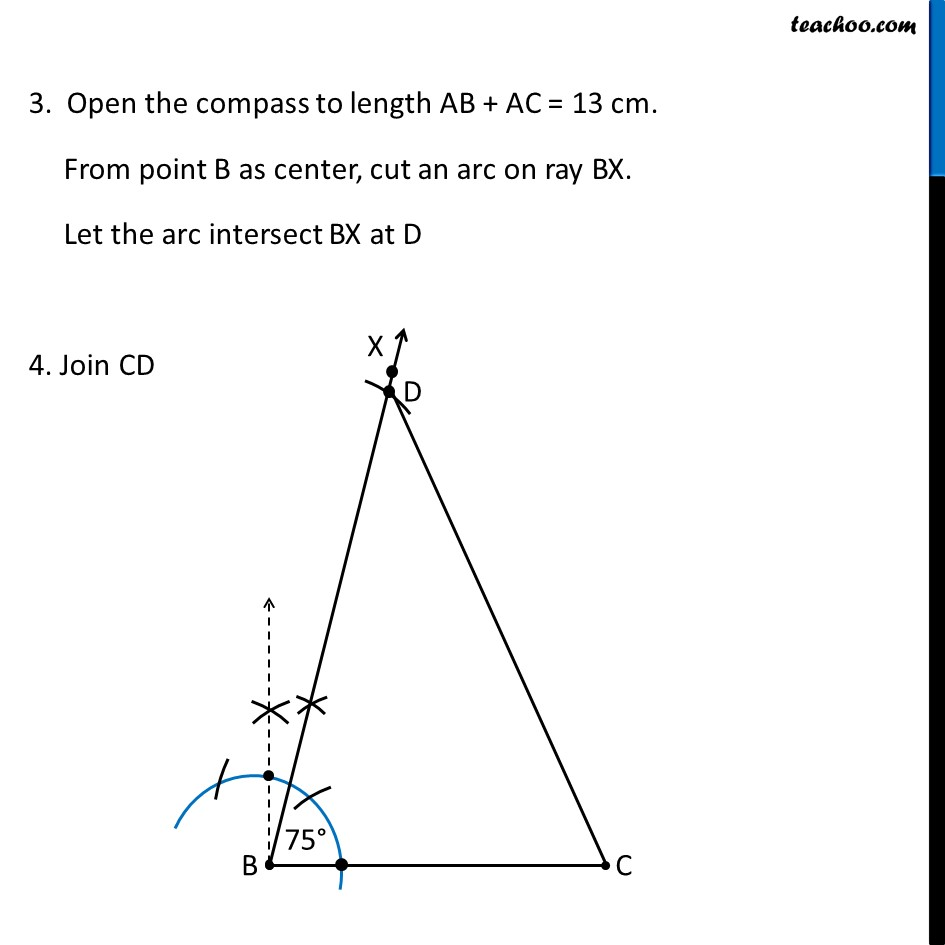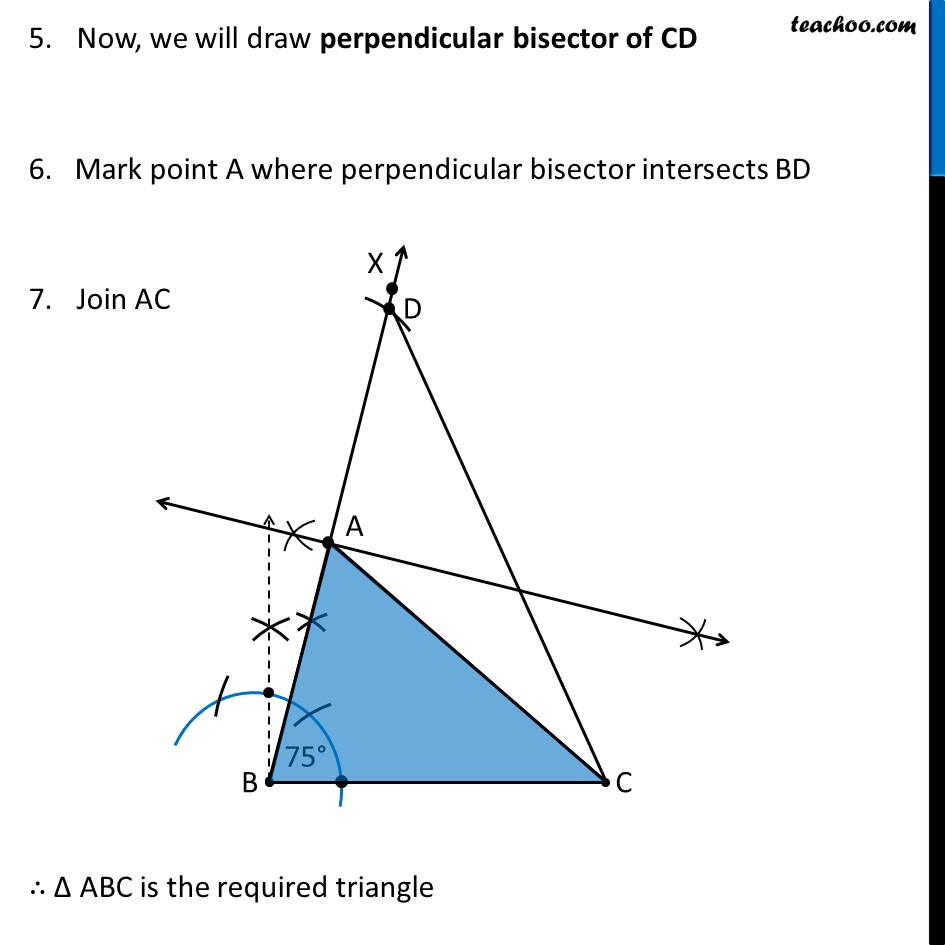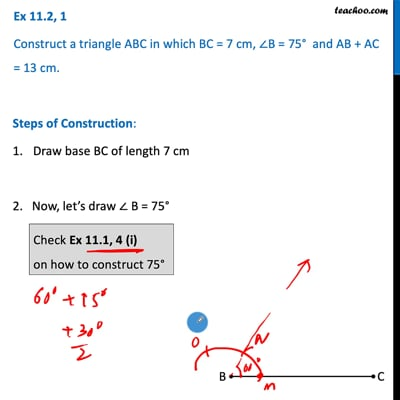Constructing Triangle - Given base, angle and sum of other 2 sidesThis video is only available for Teachoo black users

Introducing your new favourite teacher - Teachoo Black, at only ₹83 per month

### Transcript

Ex 11.2, 1 Construct a triangle ABC in which BC = 7 cm, ∠B = 75° and AB + AC = 13 cm. Steps of Construction: Draw base BC of length 7 cm 2. Now, let’s draw ∠ B = 75° Let the ray be BX Check Ex 11.1, 4 (i) on how to construct 75° 3. Open the compass to length AB + AC = 13 cm. From point B as center, cut an arc on ray BX. Let the arc intersect BX at D 4. Join CD Now, we will draw perpendicular bisector of CD 6. Mark point A where perpendicular bisector intersects BD Join AC ∴ Δ ABC is the required triangle Now, we will draw perpendicular bisector of CD 6. Mark point A where perpendicular bisector intersects BD Join AC ∴ Δ ABC is the required triangle